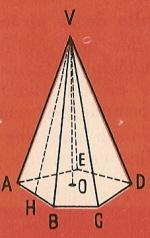Area and volume of the pyramid to printName: ______________________________________  Subject: _______________________ Date: _______

Write on the right side what is missing.

1. Pyramid parts

The Egyptian pyramids are famous.
The face that rests on the floor is the base.
Its lateral faces are Triangles which have a common vertex that is the vertex of the pyramid.
The height of the pyramid is the perpendicular segment of the apex above the base.
The apothem of a regular pyramid is the height of one of the lateral triangles.Look at this figure and answer:

 The segment VD is... The segment VO is... The segment VH is... The segment CD is...2. – Surface and lateral area of a pyramid

This square pyramid has as base a square of 18 m side. The apothem is 30 meters and we want to know the lateral area and the surface area.
We have 3 triangles of 18 m base by 30 heights. The area of a triangle is base x height: 2, that is, 18 x 30: 2 = 270 m2, as there are 4 triangles, the lateral area is 270 m2 x 4 = 1080 m2.
The lateral area can also be calculated by multiplying the perimeter of the base by apothem divided into 2. = Lateral area = base perimeter (72 m) x apothem (30) = 1080 m2.

The surface area is the sum of the lateral area plus the area of the base: 1080 + 324 = 1404 m2.

Do these problems on a paper and answer:

 What is the lateral area of a regular triangular pyramid if the side of the triangle is 14 m and the apothem of the pyramid 17 m? Find the lateral area in m2 of a regular pentagonal pyramid, being 2.61 m the side of the base and 8.25 dm the apothem of the pyramid. Calculate the surface area in dm2 of the regular square pyramid, being 7.3 dm the side of the base and 9.15 dm the apothem. What is the lateral area of a regular triangular pyramid in m2 if the side of the triangle is 20 m and the apothem 17.5 meters?3. Volume of the pyramid

In the picture, we see a pyramid P that has the same base and the same height as the prism P'. It is needed to pour 3 times the pyramid filled with sand to fill the prism. Then the volume of the pyramid is 3 times less than the prism.
The volume of the prism is the product of the base area time the height.
The volume of the pyramid will be: base area x height divided by 3.
The volume of a pyramid is the product of one third of the area of the base times height.

Do these problems on a paper and answer:

 1. Find the volume in m3 of the Great Pyramid of Cheops in Egypt whose base is a square of 230 m side, and its height is 7/10 of that side. 2. Find the volume in m3 of a regular pyramid whose base is a square of 16.7 m side, being 15 m height. 3. What is the height of a pyramid whose volume is 6.75 m3 and the base area is 15 m2? 4. What is the base area in cm2 of a pyramid of 10.92 cm3 and 7.2 cm height?

| Educational applications | Mathematics |
In Spanish  | Interactive

®Arturo Ramo García.-Record of intellectual property of Teruel (Spain) No 141, of 29-IX-1999
Plaza Playa de Aro, 3, 1ş DO 44002-TERUEL